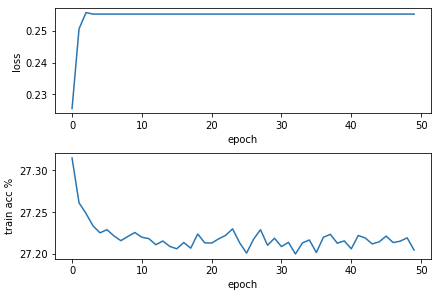# LSTM plateauing at ~25% accuracy on train set (Tensorflow version achieves > 95% test acc)

I am working on porting an effective model from TensorFlow to PyTorch but have been unable to get the network to learn effectively in PyTorch. I suspect there is a simple misunderstanding on my end of how PyTorch operates. I have been working on this port too long now and am finally willing to admit I could use a little helpThe problem I am experiencing is the train accuracy seems to peak at ~25% which is barely better than guessing. I have tried playing with the optimizer (various optimizers, learning rates, and other hyperparams) but, again, the tensorflow version does very well (so I don’t believe retuning is necessary). This leads me to believe the way I am using the optimizer is erroneous. I did confirm the model weights change on optimizer.step() by inspecting the LSTM layer’s parameters (see the training logic below).

The goal is to classify single-channel (one feature) time-series data into one of five classes.

Here is my module implementation

``````class Discriminator(nn.Module):
def __init__(self, hidden_size: int, target_seq_len: int, num_classes: int):#, batch_size: int):
super(Discriminator, self).__init__()
#self.batch_size = batch_size
self.hidden_size = hidden_size
self.num_layers = 2
self.n_units = 8
self.bidirectional = True
self.device = torch.device('cuda' if torch.cuda.is_available() else 'cpu')
drop_out_pct = 0.1
self.lstm = nn.LSTM(input_size=target_seq_len, num_layers=self.num_layers, hidden_size=hidden_size,
batch_first=True, bidirectional=self.bidirectional, dropout=0.0).to(self.device)
self.drop_out = nn.Dropout(drop_out_pct).to(self.device)
self.linear = nn.Linear(hidden_size * (2 if self.bidirectional else 1), self.n_units).to(self.device)
self.relu = nn.ReLU().to(self.device)
self.linear_out = nn.Linear(self.n_units, num_classes).to(self.device)
#self.softmax = nn.LogSoftmax(dim=1).to(self.device)
#self.init_lstm_memory() # dont do this here - we now do it every epoch

def init_lstm_memory(self, batch_size: int) -> torch.Tensor:
hidden = (
torch.zeros(((2 if self.bidirectional else 1) * self.num_layers, batch_size, self.hidden_size), dtype=torch.float).to(self.device),
torch.zeros(((2 if self.bidirectional else 1) * self.num_layers, batch_size, self.hidden_size), dtype=torch.float).to(self.device)
)

#for i, tensor in enumerate(hidden):
#  nn.init.normal_(tensor, mean=0, std=1)

return hidden

def forward(self, input: torch.Tensor, hidden: torch.Tensor) -> (torch.Tensor, torch.Tensor):
x = input.to(self.device)

x, hidden = self.lstm(x, hidden)
x = x[:, -1, :] # we're interested in the final output of the lstm

#if self.bidirectional:
#  x = torch.cat((hidden[-2, :, :], hidden[-1, :, :]), dim=1)
#else:
#  x = hidden[-1, :, :]

#print(f'shape after dropout {x.shape}')
x = self.linear(x)
x = self.relu(x)
x = self.drop_out(x)
#print(f'shape after linear {x.shape}')
x = self.linear_out(x)
#print(f'shape after linear_out {x.shape}')
#x = self.softmax(x)
x = torch.nn.functional.softmax(x, dim=1)
return x, hidden
``````

Here is my training logic

``````def repackage_hidden(h):
""" Wraps hidden states in new Tensors, to detach them from their history.
Copied from https://github.com/pytorch/examples/blob/master/word_language_model/main.py """
if isinstance(h, torch.Tensor):
return h.detach()
else:
return tuple(repackage_hidden(v) for v in h)

def trainLSTM(data: np.array, lbls: np.array):
data = np.expand_dims(data, axis=2)
print(f'data shape {data.shape}')

num_epochs = 1600
batch_size = 128
device = torch.device('cuda' if torch.cuda.is_available() else 'cpu')
label_encoder = LabelEncoder()
lbls = label_encoder.fit_transform(lbls)
x_train, x_test, y_train, y_test = train_test_split(data, lbls, stratify=lbls, test_size=0.15)
x_train = torch.from_numpy(x_train).float()#.to(device) # we dont want to put all of this on the GPU at once - at least I dont think so..
x_test = torch.from_numpy(x_test).float()#.to(device)
y_train = torch.from_numpy(y_train)#.int()#.to(device)
y_test = torch.from_numpy(y_test)#.int()#.to(device)

#print(y_test)

model = Discriminator(hidden_size=32, target_seq_len=1, num_classes=5)#, batch_size)
optimizer = RMSprop(model.parameters(), lr=LR, alpha=ALPHA) # pretty sure alpha is equivalent to TFs rho
#optimizer = SGD(model.parameters(), lr=LR, momentum=MOMENTUM)
scheduler = LambdaLR(optimizer, lambda epoch: 1.0 if epoch < 10 else math.exp(-0.1))
loss_fn = nn.CrossEntropyLoss() #nn.NLLLoss()
print(f'model params: {model.parameters()}')

train_dataset = MyDataset(x_train, y_train) # Note: MyDataset provides defs for __len__ and__getitem__ (it is trivial and the definition has been intentionally omitted in this post)
test_dataset = MyDataset(x_test, y_test)
train_dataloader = DataLoader(train_dataset, batch_size=batch_size, shuffle=True)#, drop_last=True) # drop last so we can use the same hidden state

model.train()

#print(torchinfo.summary(model))

# plot sanity visualizations - Note: this yielded the expected results
#rand_indices = random.sample(range(x_train.shape), 5)
#print(rand_indices)
#fig, axs = plt.subplots(nrows=5)
#fig.suptitle('None')
#x_vals = np.arange(longest_seq_len)
#for i in range(len(rand_indices)):
#  #print(x_train[rand_indices[i]].shape)
#  sns.lineplot(x=x_vals, y=x_train[rand_indices[i]].squeeze().numpy(), ax=axs[i])
#plt.show()
#return

for i in range(num_epochs):
print(f'epoch: {i}')
epoch_loss = epoch_acc = 0
#lstm_hidden = model.init_lstm_memory(batch_size)
#print(train_batch.shape, train_lbls.shape)
train_batch, train_lbls = train_batch.to(device), train_lbls.to(device)

#lstm_hidden = repackage_hidden(lstm_hidden)

lstm_hidden = model.init_lstm_memory(train_batch.shape)

preds, lstm_hidden = model(train_batch, lstm_hidden)

loss = loss_fn(preds, train_lbls)
#print(f'loss shape {loss.shape}')
loss.backward()

# check lstm weights
#print(next(model.lstm.parameters()))
#old_lstm_weights = list(model.lstm.parameters()).clone()

optimizer.step()

#new_lstm_weights = list(model.lstm.parameters()).clone()

#for param in model.parameters():
#return

#print(f'weights unchanged: {torch.equal(old_lstm_weights.data, new_lstm_weights.data)}')
#if torch.equal(old_lstm_weights.data, new_lstm_weights.data):
#  for param in model.parameters():
#    if param.grad is not None:
#      break

#preds = torch.nn.functional.softmax(preds, dim=1)
#print(f'first pred: {preds}')
_, preds = torch.max(preds, dim=1) # extract the predicted labels (index of max arg)

epoch_loss += loss.detach().item() # update loss
epoch_acc += (preds == train_lbls).float().sum() # update correct count

scheduler.step()
epoch_acc /= len(train_dataset) # TODO: this is an incorrect divisor if drop_last is true in the train dataloader
print(f'epoch loss: {epoch_loss:.3f}')
print(f'epoch acc: {epoch_acc:.3f}')
``````

Does anyone see anything wrong with my training loop or model which may explain the horrible results I am receiving? Any help would be greatly appreciated. Thank you for your time.

`nn.CrossEntropyLoss` expects raw logits as the model output, while you are applying a `softmax` as the last activation output. Remove it and retrain your model.

@ptrblck, thank you for your suggestion. I commented out the call to softmax in my model’s forward function but I am still receiving results which suggest no learning is occurring.The attached plot illustrates loss and training set accuracy over the first 50 epochs.

I don’t know what might be causing the issue as I’m able to overfit random data using your model and this code:

``````N = 64
data = torch.randn(N, 10, 1)
device = torch.device('cuda' if torch.cuda.is_available() else 'cpu')

model = Discriminator(hidden_size=32, target_seq_len=1, num_classes=5)
loss_fn = nn.CrossEntropyLoss()
model.train()

train_batch = data
train_lbls = torch.randint(0, 5, (N,)).to(device)

for i in range(1000):